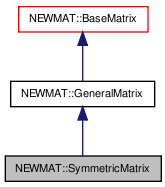# NEWMAT::SymmetricMatrix Class Reference

`#include <newmat.h>`

Inheritance diagram for NEWMAT::SymmetricMatrix:[legend]

## Detailed Description

Definition at line 667 of file newmat.h.

List of all members.

## Public Member Functions

SymmetricMatrix ()
~SymmetricMatrix ()
SymmetricMatrix (ArrayLengthSpecifier)
SymmetricMatrix (const BaseMatrix &)
void operator= (const BaseMatrix &)
SymmetricMatrixoperator= (Real f)
SymmetricMatrixoperator= (const SymmetricMatrix &m)
Real & operator() (int, int)
Real & element (int, int)
Real operator() (int, int) const
Real element (int, int) const
MatrixType Type () const
SymmetricMatrix (const SymmetricMatrix &gm)
Real SumSquare () const
Real SumAbsoluteValue () const
Real Sum () const
Real Trace () const
void GetRow (MatrixRowCol &)
void GetCol (MatrixRowCol &)
void GetCol (MatrixColX &)
void RestoreCol (MatrixRowCol &)
void RestoreCol (MatrixColX &)
GeneralMatrixTranspose (TransposedMatrix *, MatrixType)
void ReSize (int)
void ReSize (const GeneralMatrix &A)
void operator+= (const SymmetricMatrix &M)
void operator-= (const SymmetricMatrix &M)
void operator+= (Real f)
void operator-= (Real f)
void swap (SymmetricMatrix &gm)

## Private Member Functions

GeneralMatrixImage () const

## Constructor & Destructor Documentation

 NEWMAT::SymmetricMatrix::SymmetricMatrix ( )

Definition at line 671 of file newmat.h.

Referenced by Image().

 NEWMAT::SymmetricMatrix::~SymmetricMatrix ( )

Definition at line 672 of file newmat.h.

 NEWMAT::SymmetricMatrix::SymmetricMatrix ( ArrayLengthSpecifier n )

Definition at line 55 of file newmat4.cpp.

 NEWMAT::SymmetricMatrix::SymmetricMatrix ( const BaseMatrix & M )

Definition at line 121 of file newmat4.cpp.

 NEWMAT::SymmetricMatrix::SymmetricMatrix ( const SymmetricMatrix & gm )

Definition at line 687 of file newmat.h.

## Member Function Documentation

 Real NEWMAT::SymmetricMatrix::element ( int m, int n ) const

Definition at line 684 of file newmat6.cpp.

 Real & NEWMAT::SymmetricMatrix::element ( int m, int n )

Definition at line 675 of file newmat6.cpp.

 void NEWMAT::SymmetricMatrix::GetCol ( MatrixColX & mrc ) ` [virtual]`

Implements NEWMAT::GeneralMatrix.

Definition at line 470 of file newmat3.cpp.

 void NEWMAT::SymmetricMatrix::GetCol ( MatrixRowCol & mrc ) ` [virtual]`

Implements NEWMAT::GeneralMatrix.

Definition at line 431 of file newmat3.cpp.

 void NEWMAT::SymmetricMatrix::GetRow ( MatrixRowCol & mrc ) ` [virtual]`

Implements NEWMAT::GeneralMatrix.

Definition at line 398 of file newmat3.cpp.

 GeneralMatrix * NEWMAT::SymmetricMatrix::Image ( ) const` [private, virtual]`

Reimplemented from NEWMAT::GeneralMatrix.

Definition at line 767 of file newmat4.cpp.

 Real NEWMAT::SymmetricMatrix::operator() ( int m, int n ) const

Definition at line 150 of file newmat6.cpp.

 Real & NEWMAT::SymmetricMatrix::operator() ( int m, int n )

Definition at line 38 of file newmat6.cpp.

 void NEWMAT::SymmetricMatrix::operator+= ( Real f )

Reimplemented from NEWMAT::GeneralMatrix.

Definition at line 702 of file newmat.h.

 void NEWMAT::SymmetricMatrix::operator+= ( const SymmetricMatrix & M )

Definition at line 700 of file newmat.h.

 void NEWMAT::SymmetricMatrix::operator-= ( Real f )

Reimplemented from NEWMAT::GeneralMatrix.

Definition at line 703 of file newmat.h.

 void NEWMAT::SymmetricMatrix::operator-= ( const SymmetricMatrix & M )

Definition at line 701 of file newmat.h.

 SymmetricMatrix& NEWMAT::SymmetricMatrix::operator= ( const SymmetricMatrix & m )

Definition at line 677 of file newmat.h.

 SymmetricMatrix& NEWMAT::SymmetricMatrix::operator= ( Real f )

Reimplemented from NEWMAT::GeneralMatrix.

Definition at line 676 of file newmat.h.

 void NEWMAT::SymmetricMatrix::operator= ( const BaseMatrix & X )

Definition at line 387 of file newmat6.cpp.

Referenced by operator=().

 void NEWMAT::SymmetricMatrix::ReSize ( const GeneralMatrix & A ) ` [virtual]`

Reimplemented from NEWMAT::GeneralMatrix.

Definition at line 320 of file newmat4.cpp.

 void NEWMAT::SymmetricMatrix::ReSize ( int nr )

Definition at line 260 of file newmat4.cpp.

Referenced by ReSize().

 void NEWMAT::SymmetricMatrix::RestoreCol ( MatrixColX & mrc ) ` [virtual]`

Reimplemented from NEWMAT::GeneralMatrix.

Definition at line 512 of file newmat3.cpp.

 void NEWMAT::SymmetricMatrix::RestoreCol ( MatrixRowCol & ) ` [virtual]`

Reimplemented from NEWMAT::GeneralMatrix.

Definition at line 695 of file newmat.h.

 Real NEWMAT::SymmetricMatrix::Sum ( ) const` [virtual]`

Reimplemented from NEWMAT::GeneralMatrix.

Definition at line 415 of file newmat8.cpp.

 Real NEWMAT::SymmetricMatrix::SumAbsoluteValue ( ) const` [virtual]`

Reimplemented from NEWMAT::GeneralMatrix.

Definition at line 399 of file newmat8.cpp.

 Real NEWMAT::SymmetricMatrix::SumSquare ( ) const` [virtual]`

Reimplemented from NEWMAT::GeneralMatrix.

Definition at line 386 of file newmat8.cpp.

 void NEWMAT::SymmetricMatrix::swap ( SymmetricMatrix & gm )

Definition at line 704 of file newmat.h.

Referenced by NEWMAT::swap().

 Real NEWMAT::SymmetricMatrix::Trace ( ) const` [virtual]`

Reimplemented from NEWMAT::BaseMatrix.

Definition at line 558 of file newmat8.cpp.

 GeneralMatrix * NEWMAT::SymmetricMatrix::Transpose ( TransposedMatrix * , MatrixType mt ) ` [virtual]`

Reimplemented from NEWMAT::GeneralMatrix.

Definition at line 53 of file newmat5.cpp.

 MatrixType NEWMAT::SymmetricMatrix::Type ( ) const` [virtual]`

Implements NEWMAT::GeneralMatrix.

Definition at line 428 of file newmat4.cpp.

The documentation for this class was generated from the following files:

 newmat11b Generated Mon May 9 04:54:19 2016 by Doxygen 1.6.3